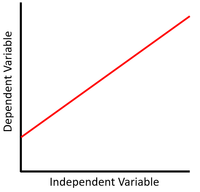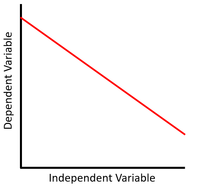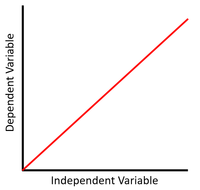# Linear Graph

(Redirected from Linear)

## Key Stage 4

### Meaning

When a relationship between two variables is linear they increase by a constant amount in relation to one another.This scatter graph shows a linear relationship where x increases and y increases. \(y = mx + c\) Where m, the gradient, is positive. This scatter graph shows a linear relationship where x increases and y decreases. \(y = mx + c\) Where m, the gradient, is negative. This scatter graph shows a linear relationship that is directly proportional where x doubles, y doubles. \(y = mx\) Where m, the gradient, is positive.